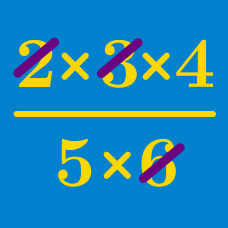Algebra

# Properties Of Arithmetic: Level 2 Challenges

$({2007} \times {20062006}) - ({2006} \times {20072007}) = \ ?$

$1 + a + 2b + 3c + 2ab + 3ac + 6bc + 6abc$

We are given that $a = \color{#D61F06}{999} , b = \color{#3D99F6}{666} , c = \color{#20A900}{333}$. Find the value of the expression above.

Hint: Try to factorize the expression.

$\large\dfrac{x^2+x^4+x^6+x^8+x^{10}}{x^2-x^4+x^6-x^8+x^{10}}=\dfrac{x^3+x^5+x^7+x^9+x^{11}}{x^3-x^5+x^7-x^9+x^{11}}$

Does the equation above hold true for all real $x\ne0$?

$4(x^2 + 2x + 1)(x^2 + 3x -2) + (x - 3)^2 \\ = (ax^2 + bx + c)^2$ If the above equation is always correct, then what is the value of $a^2 + b^2 +c^2$?

$\displaystyle \left( 1 - \frac{1}{\color{teal}{10}}\right)\left(1 - \frac{1}{\color{teal}{11}}\right)\left(1 - \frac{1}{\color{teal}{12}}\right)\cdots\left(1 - \frac{1}{\color{teal}{100}}\right)= \ ?$

×